# Connection along a curve

## Definition

Let$M$ be a differential manifold and$E$ be a vector bundle over$M$. Let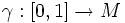$\gamma:[0,1] \to M$ be a smooth curve in$M$. A connection along$\gamma$, of$E$, is defined as follows: it is a map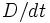$D/dt$ from the space of sections of$E$ along$\gamma$, to itself, such that: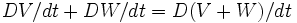$DV/dt + DW/dt = D(V + W)/dt$

and for$f:[0,1] \to \R$ we have: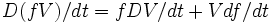$D(fV)/dt = fDV/dt + V df/dt$

where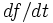$df/dt$ is usual real differentiation.

## Facts

### Connection gives connection along a curve

Given a connection on the whole vector bundle$E$, we can obtain a connection along the curve$\gamma$. Simply define: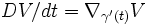$DV/dt = \nabla_{\gamma'(t)}V$

where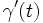$\gamma'(t)$ is the tangent vector to$\gamma$ at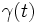$\gamma(t)$. This can also be viewed as the pullback connection for the map$\gamma$ (which we might restrict to the open interval$(0,1)$, for convenience).

However, not every connection along a curve arises from a connection. A particular case is self-intersecting curves. We can construct connections along self-intersecting curves that behave very differently for the same point at two different times, and hence cannot arise from a connection.